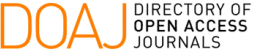### Equations of the Elastic of a Spatial Lattice from a Continuous Model

A. M. Guzmán, G. A. González del Solar, V. A. Roldán

#### Abstract

Within metal constructions there are structures made up of parallel profiles called legs, connected to each other by diagonals. Some of these structures, with a triangular cross section, turn out to be the braced masts used in the communications industry, but also columns and beams used in metal structures. In the present work, the equations that allow obtaining the equations of the elastic of a spatial lattice of triangular cross section are developed, in which the legs are joined together by zig-zag diagonals. To do this, we start from an energy proposal in which the differential equations of equilibrium and the boundary conditions of the proposed problem are determined. Finally, examples are presented where results are obtained with the equations developed, and they are compared with the results obtained from the application of the expressions given in CIRSOC 303 Recommendation (1996) and from finite element models.

#### Keywords

spatial lattice; static; strain energy; differential equations

PDF

#### References

M. Madagula, Y.Wahba, and G. Monforton, "Dynamic response of guyed mast," Engineering Structures, vol. 20, pp. 1097-1101, 1998.

Y. Wahba, M. Madagula, and G. Monforton, "Evaluation of non-linear analisis of guyed antenna towers," Computers and Structures, vol. 68, pp. 207-212, 1998.

N. B. Kahla, "Equivalent beam-column analysis of guyed towers," Computers and Structures, vol. 55, pp. 631-645, 1995.

C. Filipich and E. Bambill, "Frecuencias naturales de piezas empresilladas vía serie de potencias," Mecánica Computacional, vol. 22, 2003.

A. Guzmán, M. Rosales, and C. Filipich, "Natural vibrations and buckling of a spatial lattice structure using a continuous model derived from an energy approach," International Journal of Steel Structures, vol. 17, pp. 1-14, 2017.

A. Guzmán, M. Rosales, and C. Filipich, "Continuous one-dimensional model of a spatial lattice. deformation, vibration and buckling problems," Engineering Structures, vol. 182, pp. 290-300, 2019.

A. Guzmán and V. Roldan, "Equivalent properties for analysis as beam-column of spatial lattices of rectangular cross-section," Advanced Steel Construction, vol. 17, pp. 95-103, 2021.

CIRSOC-303, Estructuras livianas de acero. INTI, Argentina, 1996.

O. Belluzzi, Ciencia de la construcción I. Aguilera, España, 1973.

DOI: https://doi.org/10.5540/tcam.2022.024.01.00035

### Refbacks

• There are currently no refbacks.

Trends in Computational and Applied Mathematics

A publication of the Brazilian Society of Applied and Computational Mathematics (SBMAC)

Indexed in: